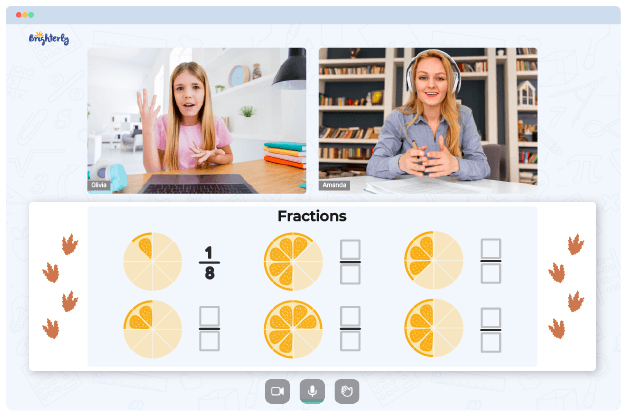# Order Fractions From Least To Greatest Worksheets

To order fractions from least to most significant, you must first find a common denominator for all of your fractions, then use the denominator to convert all the fractions so that you derive a numerator. Using the numerator, order fractions in the proper order.

This explanation may sound easy for you because you are an adult. Kids will need consistent practice to understand this concept, which is why they need an ordering fractions from least to greatest worksheet.

## Benefits of Ordering Fractions from Least to Greatest Worksheets

Kids who use an ordering fractions worksheet greatly benefit from it, and here are some of these benefits.

### Kids learn how to find the denominator

The first step to ordering fractions from the least to the greatest is finding a common denominator. Most kids can’t learn how to find the common denominator in class immediately; it requires practice. The ordering fractions from least to greatest worksheets will help them practice more.

Math for Kids

Is Your Child Struggling With Math?
1:1 Online Math Tutoring### Kids learn the correct order of fractions

Intuitively arranging fractions from the least to the greatest requires practice. The order of operations with fractions worksheet will help students get all the practice they need to know which fraction comes last and which one comes first.

### They also practice how to convert fractions to decimals

Kids first learn how to convert fractions using the ordering fractions and decimals worksheet. Afterward, they practice using the numerator to find which fractions are relatively small and which ones are big in a list.

Helping your kid with online math lessons for 4th grade. Expert tutors inspiring kids to love math so they can excel at It.

## Download an Order Fractions from Least to Greatest Worksheet in PDF### Order Fractions From Least To Greatest Worksheets PDF

Ordering Fractions From Least To Greatest Worksheet### Order Fractions From Least To Greatest Worksheets PDF

Ordering Fractions Worksheet### Order Fractions From Least To Greatest Worksheets PDF

Order Of Operations With Fractions Worksheet### Order Fractions From Least To Greatest Worksheets PDF

Ordering Fractions And Decimals Worksheet

Math websites like Brighterly have order fractions from least to greatest worksheets you can use to teach your kids. These worksheets are free and printable. So, for the best results when handling a fractions topic in your math class, download the ones with attractive visual aids; they improve engagement.

### Fractions Worksheets

Struggling with Fractions?• Does your child struggle to master of fractions?
• Try studying with an online tutor.

Is your child having trouble grasping the concept of fractions? An online tutor could provide the necessary support.